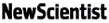|     |    |      |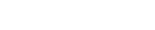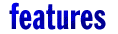## The Omega Man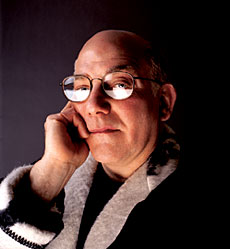Photo: Kevin KnightHe shattered mathematics with a single number. And that was just for starters, says Marcus Chown
TWO plus two equals four: nobody would argue with that. Mathematicians can rigorously prove sums like this, and many other things besides. The language of maths allows them to provide neatly ordered ways to describe everything that happens in the world around us.
Or so they once thought. Gregory Chaitin, a mathematics researcher at IBM's T. J. Watson Research Center in Yorktown Heights, New York, has shown that mathematicians can't actually prove very much at all. Doing maths, he says, is just a process of discovery like every other branch of science: it's an experimental field where mathematicians stumble upon facts in the same way that zoologists might come across a new species of primate.
Mathematics has always been considered free of uncertainty and able to provide a pure foundation for other, messier fields of science. But maths is just as messy, Chaitin says: mathematicians are simply acting on intuition and experimenting with ideas, just like everyone else. Zoologists think there might be something new swinging from branch to branch in the unexplored forests of Madagascar, and mathematicians have hunches about which part of the mathematical landscape to explore. The subject is no more profound than that.

The reason for Chaitin's provocative statements is that he has found that the core of mathematics is riddled with holes. Chaitin has shown that there are an infinite number of mathematical facts but, for the most part, they are unrelated to each other and impossible to tie together with unifying theorems. If mathematicians find any connections between these facts, they do so by luck. "Most of mathematics is true for no particular reason," Chaitin says. "Maths is true by accident."

This is particularly bad news for physicists on a quest for a complete and concise description of the Universe. Maths is the language of physics, so Chaitin's discovery implies there can never be a reliable "theory of everything", neatly summarising all the basic features of reality in one set of equations. It's a bitter pill to swallow, but even Steven Weinberg, a Nobel prizewinning physicist and author of Dreams of a Final Theory, has swallowed it. "We will never be sure that our final theory is mathematically consistent," he admits.

Chaitin's mathematical curse is not an abstract theorem or an impenetrable equation: it is simply a number. This number, which Chaitin calls Omega, is real, just as pi is real. But Omega is infinitely long and utterly incalculable. Chaitin has found that Omega infects the whole of mathematics, placing fundamental limits on what we can know. And Omega is just the beginning. There are even more disturbing numbers--Chaitin calls them Super-Omegas--that would defy calculation even if we ever managed to work Omega out. The Omega strain of incalculable numbers reveals that mathematics is not simply moth-eaten, it is mostly made of gaping holes. Anarchy, not order, is at the heart of the Universe.

Chaitin discovered Omega and its astonishing properties while wrestling with two of the most influential mathematical discoveries of the 20th century. In 1931, the Austrian mathematician Kurt Gödel blew a gaping hole in mathematics: his Incompleteness Theorem showed there are some mathematical theorems that you just can't prove. Then, five years later, British mathematician Alan Turing built on Gödel's work.

Using a hypothetical computer that could mimic the operation of any machine, Turing showed that there is something that can never be computed. There are no instructions you can give a computer that will enable it to decide in advance whether a given program will ever finish its task and halt. To find out whether a program will eventually halt--after a day, a week or a trillion years--you just have to run it and wait. He called this the halting problem.

Decades later, in the 1960s, Chaitin took up where Turing left off. Fascinated by Turing's work, he began to investigate the halting problem. He considered all the possible programs that Turing's hypothetical computer could run, and then looked for the probability that a program, chosen at random from among all the possible programs, will halt. The work took him nearly 20 years, but he eventually showed that this "halting probability" turns Turing's question of whether a program halts into a real number, somewhere between 0 and 1.

Chaitin named this number Omega. And he showed that, just as there are no computable instructions for determining in advance whether a computer will halt, there are also no instructions for determining the digits of Omega. Omega is uncomputable.

Some numbers, like pi, can be generated by a relatively short program which calculates its infinite number of digits one by one--how far you go is just a matter of time and resources. Another example of a computable number might be one that comprises 200 repeats of the sequence 0101. The number is long, but a program for generating it only need say: "repeat '01' 400 times".

There is no such program for Omega: in binary, it consists of an unending, random string of 0s and 1s. "My Omega number has no pattern or structure to it whatsoever," says Chaitin. "It's a string of 0s and 1s in which each digit is as unrelated to its predecessor as one coin toss is from the next."

The same process that led Turing to conclude that the halting problem is undecidable also led Chaitin to the discovery of an unknowable number. "It's the outstanding example of something which is unknowable in mathematics," Chaitin says.

An unknowable number wouldn't be a problem if it never reared its head. But once Chaitin had discovered Omega, he began to wonder whether it might have implications in the real world. So he decided to search mathematics for places where Omega might crop up. So far, he has only looked properly in one place: number theory.

Number theory is the foundation of pure mathematics. It describes how to deal with concepts such as counting, adding, and multiplying. Chaitin's search for Omega in number theory started with "Diophantine equations"--which involve only the simple concepts of addition, multiplication and exponentiation (raising one number to the power of another) of whole numbers.

Chaitin formulated a Diophantine equation that was 200 pages long and had 17,000 variables. Given an equation like this, mathematicians would normally search for its solutions. There could be any number of answers: perhaps 10, 20, or even an infinite number of them. But Chaitin didn't look for specific solutions, he simply looked to see whether there was a finite or an infinite number of them.

He did this because he knew it was the key to unearthing Omega. Mathematicians James Jones of the University of Calgary and Yuri Matijasevic of the Steklov Institute of Mathematics in St Petersburg had shown how to translate the operation of Turing's computer into a Diophantine equation. They found that there is a relationship between the solutions to the equation and the halting problem for the machine's program. Specifically, if a particular program doesn't ever halt, a particular Diophantine equation will have no solution. In effect, the equations provide a bridge linking Turing's halting problem--and thus Chaitin's halting probability--with simple mathematical operations, such as the addition and multiplication of whole numbers.

Chaitin had arranged his equation so that there was one particular variable, a parameter which he called N, that provided the key to finding Omega. When he substituted numbers for N, analysis of the equation would provide the digits of Omega in binary. When he put 1 in place of N, he would ask whether there was a finite or infinite number of whole number solutions to the resulting equation. The answer gives the first digit of Omega: a finite number of solutions would make this digit 0, an infinite number of solutions would make it 1. Substituting 2 for N and asking the same question about the equation's solutions would give the second digit of Omega. Chaitin could, in theory, continue forever. "My equation is constructed so that asking whether it has finitely or infinitely many solutions as you vary the parameter is the same as determining the bits of Omega," he says.

But Chaitin already knew that each digit of Omega is random and independent. This could only mean one thing. Because finding out whether a Diophantine equation has a finite or infinite number of solutions generates these digits, each answer to the equation must therefore be unknowable and independent of every other answer. In other words, the randomness of the digits of Omega imposes limits on what can be known from number theory--the most elementary of mathematical fields. "If randomness is even in something as basic as number theory, where else is it?" asks Chaitin. He thinks he knows the answer. "My hunch is it's everywhere," he says. "Randomness is the true foundation of mathematics."

The fact that randomness is everywhere has deep consequences, says John Casti, a mathematician at the Santa Fe Institute in New Mexico and the Vienna University of Technology. It means that a few bits of maths may follow from each other, but for most mathematical situations those connections won't exist. And if you can't make connections, you can't solve or prove things. All a mathematician can do is aim to find the little bits of maths that do tie together. "Chaitin's work shows that solvable problems are like a small island in a vast sea of undecidable propositions," Casti says.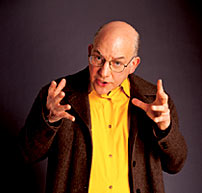Photo: Kevin KnightTake the problem of perfect odd numbers. A perfect number has divisors whose sum makes the number. For example, 6 is perfect because its divisors are 1, 2 and 3, and their sum is 6. There are plenty of even perfect numbers, but no one has ever found an odd number that is perfect. And yet, no one has been able to prove that an odd number can't be perfect. Unproved hypotheses like this and the Riemann hypothesis, which has become the unsure foundation of many other theorems (New Scientist, 11 November 2000, p 32) are examples of things that should be accepted as unprovable but nonetheless true, Chaitin suggests. In other words, there are some things that scientists will always have to take on trust.

Unsurprisingly, mathematicians had a difficult time coming to terms with Omega. But there is worse to come. "We can go beyond Omega," Chaitin says. In his new book, Exploring Randomness (New Scientist, 10 January, p 46), Chaitin has now unleashed the "Super-Omegas".

Like Omega, the Super-Omegas also owe their genesis to Turing. He imagined a God-like computer, much more powerful than any real computer, which could know the unknowable: whether a real computer would halt when running a particular program, or carry on forever. He called this fantastical machine an "oracle". And as soon as Chaitin discovered Omega--the probability that a random computer program would eventually halt--he realised he could also imagine an oracle that would know Omega. This machine would have its own unknowable halting probability, Omega'

But if one oracle knows Omega, it's easy to imagine a second-order oracle that knows Omega'. This machine, in turn, has its own halting probability, Omega'', which is known only by a third-order oracle, and so on. According to Chaitin, there exists an infinite sequence of increasingly random Omegas. "There is even an all-seeing infinitely high-order oracle which knows all other Omegas," he says.

He kept these numbers to himself for decades, thinking they were too bizarre to be relevant to the real world. Just as Turing looked upon his God-like computer as a flight of fancy, Chaitin thought these Super-Omegas were fantasy numbers emerging from fantasy machines. But Veronica Becher of the University of Buenos Aires has shown that Chaitin was wrong: the Super-Omegas are both real and important. Chaitin is genuinely surprised by this discovery. "Incredibly, they actually have a real meaning for real computers," he says.

Becher has been collaborating with Chaitin for just over a year, and is helping to drag Super-Omegas into the real world. As a computer scientist, she wondered whether there were links between Omega, the higher-order Omegas and real computers.

Real computers don't just perform finite computations, doing one or a few things, and then halt. They can also carry out infinite computations, producing an infinite series of results. "Many computer applications are designed to produce an infinite amount of output," Becher says. Examples include Web browsers such as Netscape and operating systems such as Windows 2000.

This example gave Becher her first avenue to explore: the probability that, over the course of an infinite computation, a machine would produce only a finite amount of output. To do this, Becher and her student Sergio Daicz used a technique developed by Chaitin. They took a real computer and turned it into an approximation of an oracle. The "fake oracle" decides that a program halts if--and only if--it halts within time T. A real computer can handle this weakened version of the halting problem. "Then you let T go to infinity," Chaitin says. This allows the shortcomings of the fake to diminish as it runs for longer and longer.

Using variations on this technique, Becher and Daicz found that the probability that an infinite computation produces only a finite amount of output is the same as Omega', the halting probability of the oracle. Going further, they showed that Omega'' is equivalent to the probability that, during an infinite computation, a computer will fail to produce an output--for example, get no result from a computation and move on to the next one--and that it will do this only a finite number of times.

These might seem like odd things to bother with, but Chaitin believes this is an important step. "Becher's work makes the whole hierarchy of Omega numbers seem much more believable," he says. Things that Turing--and Chaitin--imagined were pure fantasy are actually very real.

Now that the Super-Omegas are being unearthed in the real world, Chaitin is sure they will crop up all over mathematics, just like Omega. The Super-Omegas are even more random than Omega: if mathematicians were to get over Omega's obstacles, they would face an ever-elevated barrier as they confronted Becher's results.

And that has knock-on effects elsewhere. Becher and Chaitin admit that the full implications of their new discoveries have yet to become clear, but mathematics is central to many aspects of science. Certainly any theory of everything, as it attempts to tie together all the facts about the Universe, would need to jump an infinite number of hurdles to prove its worth.

The discovery of Omega has exposed gaping holes in mathematics, making research in the field look like playing a lottery, and it has demolished hopes of a theory of everything. Who knows what the Super-Omegas are capable of? "This," Chaitin warns, "is just the beginning."

 Further reading: Exploring Randomness by G. J. Chaitin, Springer-Verlag (2001) "A Century of Controversy Over the Foundations of Mathematics" by G. J. Chaitin, Complexity, vol 5, p 12 (2000) The Unknowable by G. J. Chaitin, Springer-Verlag (1999) "Randomness everywhere" by C. S. Calude and G. J. Chaitin, Nature, vol 400, p 319 (1999) http://www.cs.umaine.edu/~chaitin/

From New Scientist magazine, 10 March 2001.